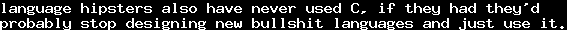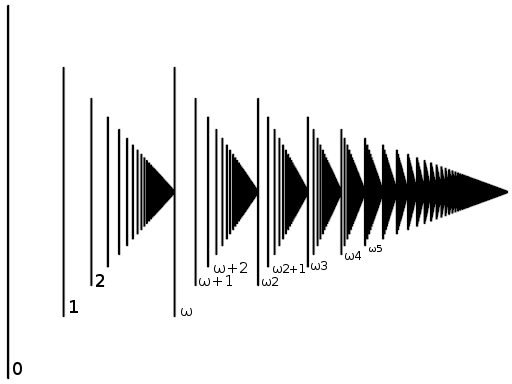# On Infinity 6 - Well-OrderingsTue, 13 Mar 2012 13:30:00 GMT

Be sure to read the former parts 1, 2, 3, 4 and especially part 5 about orderings, before you read this part.

There are a lot of people trying to tell about what mathematics or a more specific topic is actually about - and they are often right in some sense, looking at it from a certain perspective. However, mostly those perspectives are missing something. Therefore, I will not make the claim that talking about infinitary objects is talking about orderings. However, what I can say is that ordering relations play an important role.

For example, many important definitions oncan be made in terms of the default ordering, like convergence and openness.

However, there is one sort of ordering relations which especially suits the purpose of describing connections between infinitary objects: the well-orderings. We will introduce this concept here.

In part 1, we gave axioms for natural numbers. These were

• Every number has a unique successor
• Every number excepthas a predecessor
• Every non-empty set has a smallest element
We now want to generalize these. To generalize something, the axioms must be loosened. Loosening the first axiom would lead to a structures mathematicians would call trees (yes, like the plant), but we do not want to discuss these here - we keep the axiom of unique successors. We have seen that the third axiom is useful in proofs. The property is called well-foundedness. We will also keep this one. So the only thing we can loosen is the second: We will allow additional elements which have no predecessor.

Now consider the setof pairs of natural numbers. We give an orderingbyThat is, we first sort by the left element, and then, if the left elements are equal, by the right element. This is called the lexicographical order on. It is, in fact, an ordering (exercise).

This relation satisfies our two remaining axioms: The unique successor of a pairis obviously. To get the minimum of a set, we first notice thathas a minimal element, as it is a subset of, and  thenalso has a smallest element, and then,is a minimal element of. The "zero" of this ordering, that is, the smallest element, is obviously.

A further example for a pair with no predecessor is. Even though there are infinitely many pairs, namelysmaller than, none of them is the predecessor of.

Now, keep in mind that we still want a generalization of the order of the natural numbers. We can embedintousing the injective homomorphism. As mathematicians are lazy, we will now just identify the naturals by this homomorphism, that is, we will actually writeinstead of. With this inclusion,becomes the first element that is larger than all the "naturals". We will - for now - writefor, andfor, and - to make it even more confusing -for.Source: Wikipedia

In fact, we can then do addition with these new objects, and multiplication with a new object and a natural, as we did with naturals - with distributive and associative laws:However, what we cannot do yet is multiplying two of these new objects:is not defined yet. We can generalize the above concept by looking at infinite sequences of natural numbers, which end in a sequence of zeroes, that is,,, etc., and apply the lexicographical order on their reversed sequences:That is, we get for the largest indexsuch that, and use its order. Our former ordering of pairs can be embedded by.

Now,, and, and generally,, and we can write a sequenceas. This definition is only provisional: the actual definition is more complicated, especially, addition is not commutative,. Our definition works well if we always have larger numbers on the left side of the addition, and we will silently ignore the fact that we did not define it for other cases.Source:Wikipedia

Ordering relations likeare called well-orderings: A well-orderingon a setis an ordering, for which every subsethas a smallest element.

As you have seen in the examples, with well-orderings we can get beyond the tower of natural numbers, into "transfinite" numbers. However, well-orderings have a surprising property, which makes them suitable for analysis of infinity:

Letbe a well-ordering, andbe a strictly decreasing sequence of elements of. Thenis of finite length.
Proof: The set of sequence elementsmust have a smallest element. Asis strictly decreasing, after reaching this element,must end immediately.

Interestingly, we can now find an ordering relation on well-orderings: Letandbe well-orderings, then there is either an injective homomorphismor an injective homomorphismor both, when they are isomorphic - as this is a bit harder to prove, we omit the proof.

So we can find a relationbetween well-orderings, whereshall mean that there exisit an injective homomorphism. If we haveand, then the well-ordernigs are isomorphic, we writefor that.

The collectioncontains all the well-orderings isomorphic to a well-ordering, and as it contains all the relevant structural information of the ordering, it is called the ordering type of.

And now ... it gets complicated. We definedas a collection, but not as a set! The axioms of set theory require sets to obey some restrictions - and this collection does not do so. It is a so-called proper class, that is a collection which is too big to be a set. However, it is not necessary to understand the reasons right now, and so I will not give any. The important thing is that being proper classes makes ordering types pretty hard to handle, which is why mathematicians had to invent a different method. For every ordering type, they gave a representative, that is a well-ordering which is isomorphic to the others, but has an additional property that uniquely determines it. These representatives are called ordinal numbers.

While originally I wanted to discuss ordinal numbers in detail with this post, the post turns out to become longer than I expected. I think, for this time, the amount of new knowledge floating through my dear readers' brains is enough.